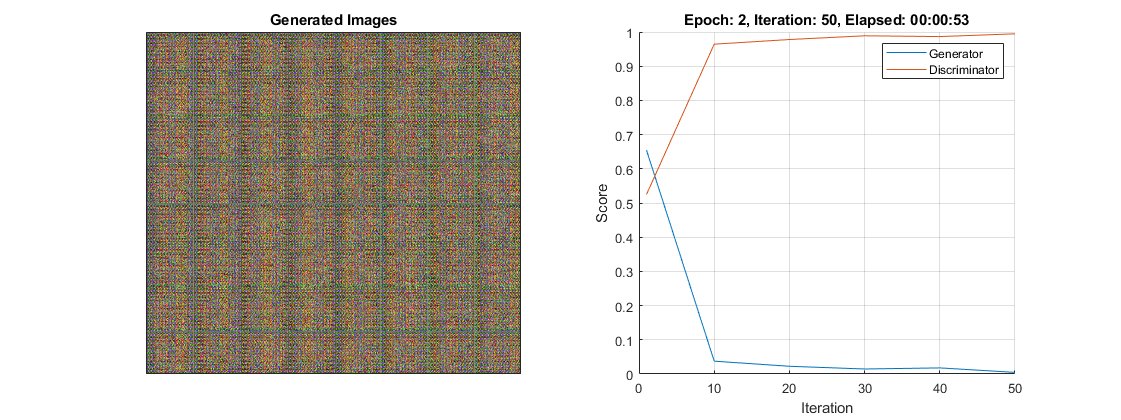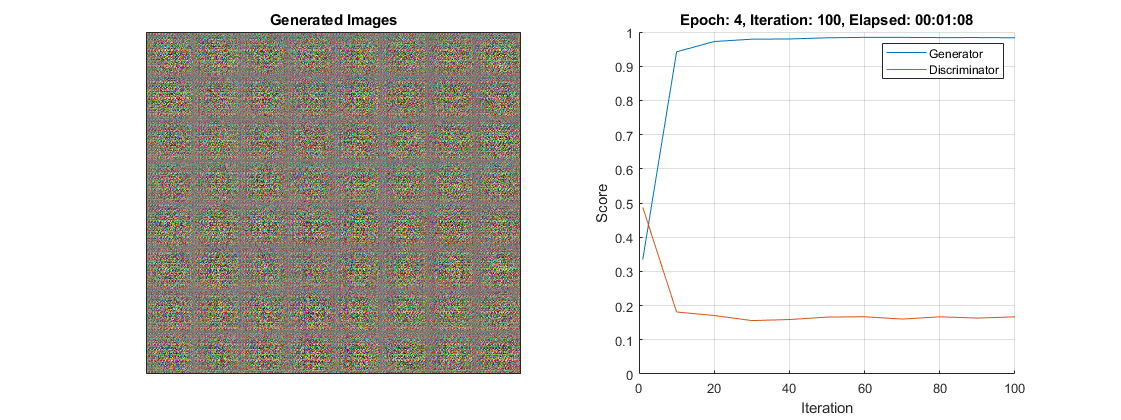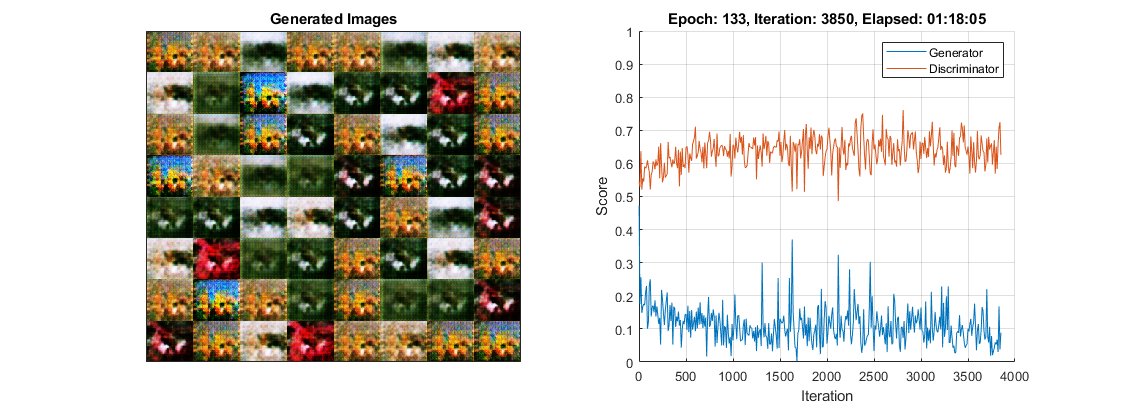Main Content

## Monitor GAN Training Progress and Identify Common Failure Modes

Training GANs can be a challenging task. This is because the generator and the discriminator networks compete against each other during the training. In fact, if one network learns too quickly, then the other network may fail to learn. This can often result in the network not being able to converge. To diagnose issues and monitor on a scale from 0 to 1 how well the generator and discriminator achieve their respective goals you can plot their scores. For an example showing how to train a GAN and plot the generator and discriminator scores, see Train Generative Adversarial Network (GAN).

The discriminator learns to classify input images as "real" or "generated". The output of the discriminator corresponds to a probability $\stackrel{^}{Y}$ that the input images belong to the class "real".

The generator score is the mean of the probabilities corresponding to the discriminator output for the generated images:

`$\text{scoreGenerator}=\text{mean}\left({\stackrel{^}{Y}}_{\text{Generated}}\right),$`

where ${\stackrel{^}{Y}}_{\text{Generated}}$ contains the probabilities for the generated images.

Given that $1-\stackrel{^}{Y}$ is the probability of an image belonging to the class "generated", the discriminator score is the mean of the probabilities of the input images belonging to the correct class:

`$\text{scoreDisriminator}=\frac{1}{2}\text{mean}\left({\stackrel{^}{Y}}_{\text{Real}}\right)+\frac{1}{2}\text{mean}\left(1-{\stackrel{^}{Y}}_{\text{Generated}}\right),$`

where ${\stackrel{^}{Y}}_{\text{Real}}$ contains the discriminator output probabilities for the real images and the numbers of real and generated images passed to the discriminator are equal.

In the ideal case, both scores would be 0.5. This is because the discriminator cannot tell the difference between real and fake images. However, in practice this scenario is not the only case in which you can achieve a successful GAN.

To monitor the training progress you can visually inspect the images over time and check if they are improving. If the images are not improving, then you can use the score plot to help you diagnose some problems. In some cases, the score plot can tell you there is no point continuing training, and you should stop, because a failure mode has occurred that training cannot recover from. The following sections tell you what to look for in the score plot and in the generated images to diagnose some common failure modes (convergence failure and mode collapse), and suggests possible actions you can take to improve the training.

### Convergence Failure

Convergence failure happens when the generator and discriminator do not reach a balance during training.

#### Discriminator Dominates

This scenario happens when the generator score reaches zero or near zero and the discriminator score reaches one or near one.

This plot shows an example of the discriminator overpowering the generator. Notice that the generator score approaches zero and does not recover. In this case, the discriminator classifies most of the images correctly. In turn, the generator cannot produce any images that fool the discriminator and thus fails to learn.If the score does not recover from these values for many iterations, then it is better to stop the training. If this happens, then try balancing the performance of generator and the discriminator by:

• Impairing the discriminator by randomly giving false labels to real images (one-sided label flipping)

• Impairing the discriminator by adding dropout layers

• Improving the generator's ability to create more features by increasing the number of filters in its convolution layers

• Impairing the discriminator by reducing its number of filters

For an example showing how to flip the labels of the real images, see Train Generative Adversarial Network (GAN).

#### Generator Dominates

This scenario happens when the generator score reaches one or near one.

This plot shows an example of the generator overpowering the discriminator. Notice that the generator score goes to one for a many iterations. In this case, the generator learns how to fool the discriminator almost always. When this happens very early in the training process, the generator is likely to learn a very simple feature representation which fools the discriminator easily. This means that the generated images can be very poor, despite having high scores. Note that in this example, the score of the discriminator does not go very close to zero because it is still able to classify correctly some real images.If the score does not recover from these values for many iterations, then it is better to stop the training. If this happens, then try balancing the performance of generator and the discriminator by:

• Improving the discriminator's ability to learn more features by increasing the number of filters

• Impairing the generator by adding dropout layers

• Impairing the generator by reducing its number of filters

### Mode Collapse

Mode collapse is when the GAN produces a small variety of images with many duplicates (modes). This happens when the generator is unable to learn a rich feature representation because it learns to associate similar outputs to multiple different inputs. To check for mode collapse, inspect the generated images. If there is little diversity in the output and some of them are almost identical, then there is likely mode collapse.

This plot shows an example of mode collapse. Notice that the generated images plot contains a lot of almost identical images, even though the inputs to the generator were different and random.If you observe this happening, then try to increase the ability of the generator to create more diverse outputs by:

• Increasing the dimensions of the input data to the generator

• Increasing the number of filters of the generator to allow it to generate a wider variety of features

• Impairing the discriminator by randomly giving false labels to real images (one-sided label flipping)

For an example showing how to flip the labels of the real images, see Train Generative Adversarial Network (GAN).

Download ebook